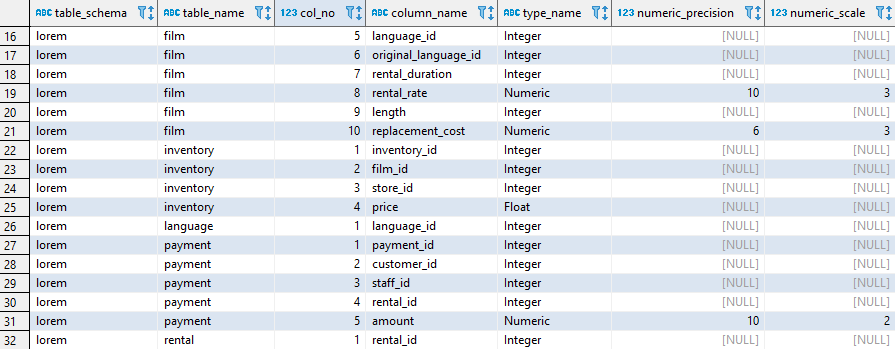## Find all numeric columns in Vertica database

Article for:

Numeric in Vertica database are columns with the following data types: Integer, Float, Numeric.

The query below lists all columns with numeric data types.

## Query

``````select table_schema,
table_name,
ordinal_position as col_no,
column_name,
t.type_name,
numeric_precision,
numeric_scale
from v_catalog.columns c
join v_catalog.types t
on c.data_type_id = t.type_id
where type_name in ('Integer', 'Float', 'Numeric')
order by table_schema,
table_name,
ordinal_position;
``````

## Columns

• table_schema - schema name
• table_name - name of the table
• col_no - column position within table
• column_name - name of the column
• type_name - type of data
• numeric_precision - precision of the numeric column
• numeric_scale - scale of the numeric column

## Rows

• One row represents one column with a numeric data type
• Scope of rows: all columns containing numeric data types in the database
• Ordered by schema name and table name

## Sample results